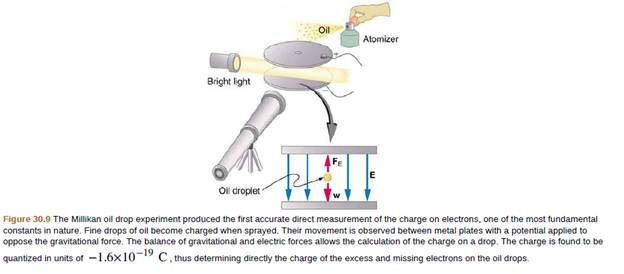Chapter 30, Problem 56PE

Chapter
Section
Textbook Problem

Integrated Concepts In a Millikan oil-drop experiment using a setup like that in Figure 30.9, a 500-V potential difference is applied to plates separated by 2.50 cm. (a) What is the mass of an oil drop having two extra electrons that is suspended motionless by the field between the plates? (b) What is the diameter of the drop, assuming it is a sphere with the density of olive oil?To determine

(a)

The mass of an oil drop having two extra electrons that is suspended motionless by the field between the plates.

Explanation

Given Data:

Given in a Millikan oil drop experiment, 500V potential difference is applied to the plates separated by 2.50cm.

Formula Used:

Force due to electric field is calculated as

FE=qE

Where FE= Force due to electric field

q= Charge

E= Electric Field

Also, electric field is calculated as

E=Vd

Where E= Electric Field

V= Voltage

d= Separation between the plates

Also, force due to gravity is calculated as

FG=mg

Where FG= Force due to gravity

m= Mass

g= Acceleration due to gravity

Calculation:

In Millikan's oil drop experiment, the oil drop is suspended between the two charged plates, such that force due to gravity and force due to electric field are in equilibrium.

Thus, we have

FG=FE

mg=qE

mg=qVd

Also, there are two extra electrons

To determine

(b)

The diameter of the drop, assuming it is a sphere with the density of olive oil.

Still sussing out bartleby?

Check out a sample textbook solution.

See a sample solution

The Solution to Your Study Problems

Bartleby provides explanations to thousands of textbook problems written by our experts, many with advanced degrees!

Get Started

What are some characteristics of the adhesive used for Post-it Notes?

General Chemistry - Standalone book (MindTap Course List)

Which is required as part of hemoglobin? iron calcium vitamin C potassium

Nutrition: Concepts and Controversies - Standalone book (MindTap Course List)

What is the relationship between an exon and a gene?

General, Organic, and Biological Chemistry